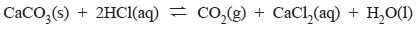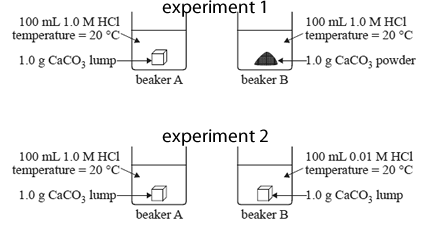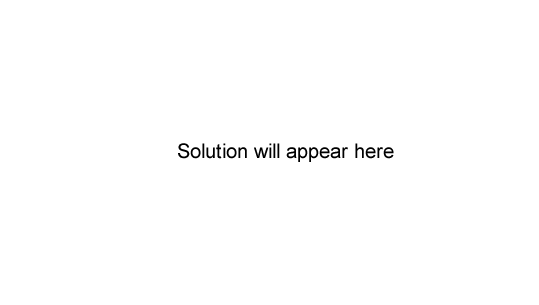Rates of reaction (2012 VCE) 1) Two experiments were conducted to investigate various factors that affect the rate of reaction between calcium carbonate and dilute hydrochloric acid.The two experiments are summarised in the diagrams below.a) How could the rate of this reaction be measured in these experiments? Solutionb) Identify the rate determining factor that is investigated in experiment 1. Solution c) In experiment 2, will the rate of reaction be faster in beaker A or beaker B? Explain your selection in terms of collision theory. Solutiond) Why is the following statement incorrect? 'Collision theory states that all collisions between reactant particles will result in a chemical reaction.' Solution